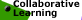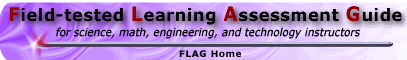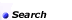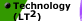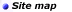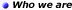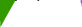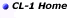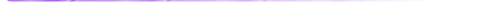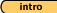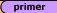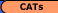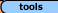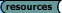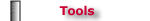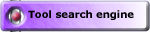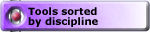## Tools - Math 'Convincing and Proving' Always, Sometimes or Never True Tasks, Set #2 (solutions)

Always, Sometimes or Never True: Set #1 (solutions) || Set #2 (solutions)
Critiquing 'Proofs': Set #3 (solutions) || Set #4 (solutions)

Malcolm Swan
Mathematics Education
University of Nottingham
Malcolm.Swan@nottingham.ac.uk

Jim Ridgway
School of Education
University of Durham
Jim.Ridgway@durham.ac.uk

Introduction:
You will be given a number of statements. You must decide if each statement is

• always true, or
• sometimes true, or
• never true
You must provide full and convincing reasons for your decision. If you think that a statement is sometimes true, you must fully explain when it is true and when it is not true.

Here is an example of what we mean:

 Example: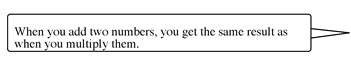Weaker response: This statement is sometimes true. It is true when both numbers are 0 and when both numbers are 2. It is not true when one number is 2 and one number is 3. Stronger response: This statement is sometimes true. Suppose one number is x and one number is y. The statement says that: x+y = xy This simplifies to the condition that y = x/(x-1) A few pairs of numbers when it works are therefore: (0, 0); (2, 2); (3, 3/2); (4, 4/3); (5, 5/4) ...... There are also other pairs which work!

The aim of this assessment is to provide the opportunity for you to:

• test statements to see how far they are true;
• provide examples or counterexamples to support your conclusions
• provide convincing arguments or proofs to support your conclusions
For each statement, say whether it is always, sometimes or never true.
You must provide several examples or counterexamples to support your decision.
Try also to provide convincing reasons for your decision.
You may even be able to provide a proof in some cases.

 1. The center of a circle that circumscribes a triangle is inside the triangle.

Is this always, sometimes or never true? ......................................................

Reasons or examples:

Sample Solution: Sometimes true.

The center of the circle that circumscribes a triangle sometimes lies inside the triangle. The statement is true when the triangle is acute. In the case of a right triangle the center of the circumscribing circle lies on the triangle. In the case of an obtuse triangle, the center of the circumscribing triangle lies outside the triangle.

 2. An altitude subdivides a triangle into two similar triangles.

Is this always, sometimes or never true? ......................................................

Reasons or examples:

Sample Solution: Sometimes true.

It is sometimes true that an altitude subdivides a triangle into similar triangle. Two interesting case are that of an altitude drawn in an isosceles triangle from the non-base angle, and that of an altitude drawn in a right triangle from the right angle.

 3. (a + b)2 = a2 + b2

Is this always, sometimes or never true? ......................................................

Reasons or examples:

Sample Solution: Sometimes true.

This is only true when a = 0 or b = 0 or a = b = 0.

 4. 3x2 = (3x)2

Is this always, sometimes or never true? ......................................................

Reasons or examples:

Sample Solution: Sometimes true.

Again this is true only when x = 0

 5. A shape with a finite area has a finite perimeter.

Is this always, sometimes or never true? ......................................................

Reasons or examples:

Sample Solution: Sometimes true.

If the shape has fixed area A and dimensions x and A/x. The perimeter is thus 2(x + A/x). As x is varied, this can be made as large or as small as we please. So theoretically, the perimeter may become infinite - but then is an infinitely thin rectangle still a rectangle (it feels more like a line to me!)? As an aside, it may also be noted that many fractals also have infinite boundaries but enclose finite areas. Moving up a dimension, it is also interesting to note that the trumpet shape obtained by rotating the curve y = 1/x around the x axis for x > 1 has a finite volume, but an infinite surface area. (Does this suggest that we can fill the shape with a finite amount of paint, but that the paint will never fully coat the surface?)

 6. A shape with a finite perimeter has a finite area.

Is this always, sometimes or never true? ......................................................

Reasons or examples:

Sample Solution: Always true.

It is always true that a shape with a finite perimeter has a finite area. If the dimensions of the rectangle are x and y, then if 2(x + y) is finite and x and y are both positive, both x and y must be finite, so the area must be finite.

Always, Sometimes or Never True: Set #1 (solutions) || Set #2 (solutions)
Critiquing 'Proofs': Set #3 (solutions) || Set #4 (solutions)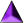Introduction || Assessment Primer || Matching Goals to CATs || CATs || Tools || Resources

Search || Who We Are || Site Map || Meet the CL-1 Team || WebMaster || Copyright || Download
College Level One (CL-1) Home || Collaborative Learning || FLAG || Learning Through Technology || NISE